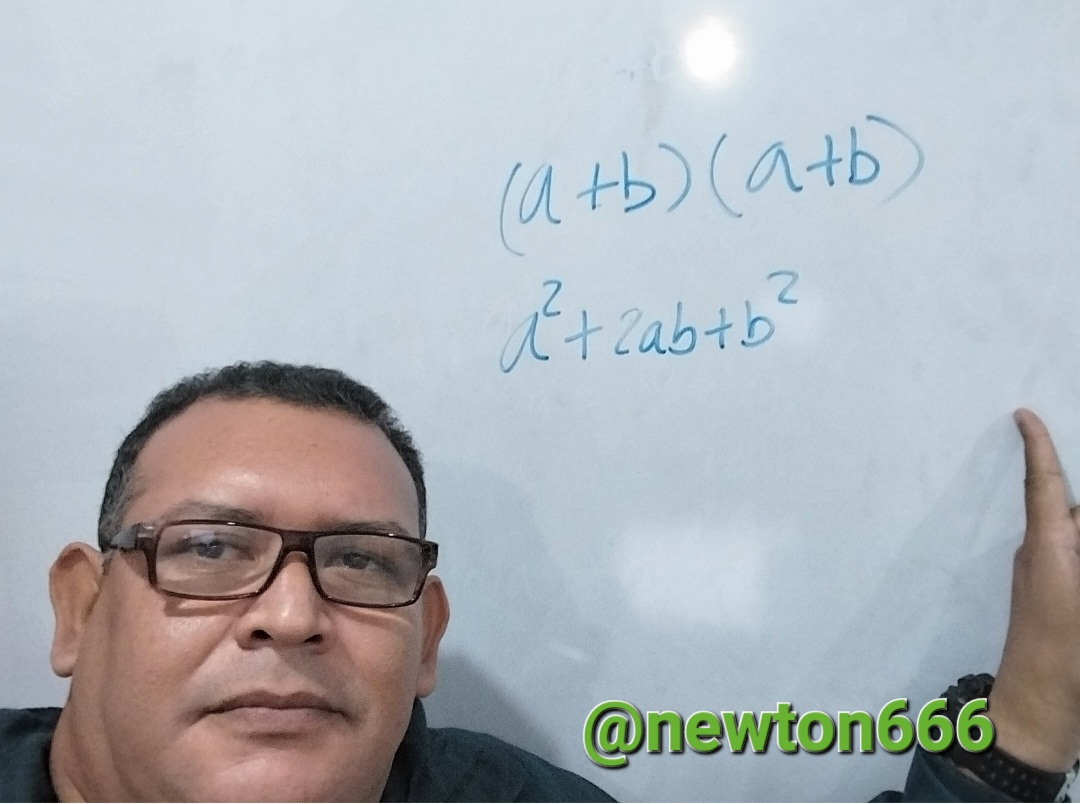# Kinetic energy using remarkable product

in StemSocial3 months agoGreetings to all science enthusiasts! Today, I'd like to share with you a remarkable product equation that is used to calculate kinetic energy in the field of physics. Since mathematical equations are essential tools for problem solving and comprehending the world around us, I hope you find this equation to be both fascinating and useful. The so-called "remarkable product" is one of the most significant mathematical equations because it makes it easier to multiply complicated algebraic expressions. This post will examine the amazing product equation and the ways in which it can be used to resolve mathematical issues.

The multiplication of two algebraic formulas of the form (a + b) * (a + b) is made simpler using the amazing product equation. The formula for this equation is a2 + 2ab + b2. The distributive of multiplication over addition, which tells us that (a + b) * c = a * c + b * c, is the source of this expression. This property is applied twice to produce the astounding product equation.

In arithmetic issues involving the multiplication of algebraic expressions, this equation is frequently utilized. As an illustration, we may utilize the noteworthy product equation to simplify multiplication if we have the statement (3x + 4y) * (3x + 4y). We start by determining that a = 3x and b = 4y. Then, we use the extraordinary product formula to get:

(3x)^2 + 2(3x)(4y) + (4y)^2

= 9x^2 + 24xy + 16y^2

By doing this, we can make the original expression more approachable.

Algebraic expressions can be factored using the famous product equation. We can factor an expression of the form (a + b)2 if it has the formula a2 + 2ab + b2. Since it enables us to simplify the expression and make it simpler to locate the answers, this is helpful in problems where we are requested to find the roots of an algebraic equation.

An essential mathematical technique for making algebraic statements easier to multiply and factor is the noteworthy product equation. We can solve math problems more quickly and accurately with your assistance. Thus, it is crucial that math students have a solid understanding of this equation and how to use it to solve problems.

It is possible to determine the kinetic energy of an item in motion using the remarkable product equation from the discipline of physics.

A given object's kinetic energy (KE) is equal to half of its mass (m) times the square of its velocity (v):KE = 1/2 * m * v^2

We can rewrite this equation using the notable product formula for the square of a binomial:

v^2 = (v + v/2)^2 = v^2 + 2v(v/2) + (v/2)^2

Simplifying the equation we get:

v^2 = v^2 + v^2 + v^2/4

Therefore:

KE = 1/2 * m * (v^2 + v^2 + v^2/4)

KE = 3/4 * m * v^2

We can therefore modify the kinetic energy equation to represent it in terms of the squared velocity of an object and its mass using the remarkable product equation.

Photo edited by my Samsung A23 phone

Bibliography Reference

From arithmetic to algebra by Salazar Guerrero, Ludwig.

Factorization by Francisco G. Mejía Duque, 2006.Sort:Thanks for your contribution to the STEMsocial community. Feel free to join us on discord to get to know the rest of us!

Please consider delegating to the @stemsocial account (85% of the curation rewards are returned).

Thanks for including @stemsocial as a beneficiary, which gives you stronger support.